# Important Feature Transformation Techniques in Machine Learning | Hands-on Feature Engineering Part-III

Welcome to part-3 of our hands-on feature engineering series. Feature engineering plays a very vital role in model performance. It is been said that if you good bad data to very optimized model and good data to bad model then the bad model will outperform as compared to the optimized model. The complete game of performance and building a well-generalized model depends on how you explore and prepare the data and this is a reason why 80 percent of the time data scientist gives to data preparation in any machine learning project. In this blog, we will discuss different feature transformation techniques that you can apply to the features of your dataset to generate more insights.

### Introduction to feature transformation

Feature Transformation is basically a technique that helps you to convert the skewed distribution of your variable into a normal distribution to help your model work better with a great performance. If you are familiar with some machine learning algorithms or not also then machine learning algorithms work very well when data is normally distributed. It is because most real-life examples are based on a normal distribution. The skewed distribution is of two types left-skewed and right-skewed data### Why Feature Transformation is required?

Feature transformation is one of the crucial steps in the feature engineering process which helps to improve model performance because most of the Machine learning models like linear models, naive Bayes generally assume that the data is normally distributed(symmetrical shape), But the data we got from the real world scenario is not normally distributed, indeed it's skewed (left-skewed or right-skewed).

• A normal distribution is a bell-shaped curve that explains that data is normally distributed or data is centered across the mean. In normal distribution mean, median, and model are approximately equal.
• Left skewed means there is a long tail towards the left side(negative axis) and data is at a peak on the right side. For example, there are very few students who got below D or E grade, and most of the students, are average and above average. This mode is greater than the median which is greater than the mean.
• Right skewed distribution means there is a long tail towards the right side (positive axis). A very good example of it is there are very few people with maximum wealth and a large number of people are in between or below that. In this mean is greater than median which is greater than mode.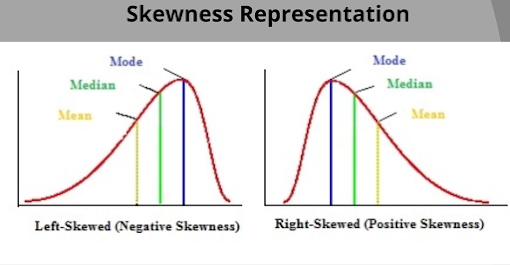So by applying the various transformation techniques we can transform the skewed distribution to the normal distribution of a variable that could help us to implement a generalized model with a decent performance.

### How to Check Distribution of any variable?

Before applying a transformation technique to a particular variable we need to identify a distribution of a variable and this can simply be done using a Histogram (distribution plot) or QQ plot (Probability plot). These plots can simply be made using a visualization library a Matplotlib or Seaborn. So without any more waste head over to the coding environment and download the dataset to practice each technique.

#### Overview of sample dataset

The dataset we are going to use a very famous titanic dataset that we are using from the past 2 blogs in the feature engineering series to demonstrate each technique. If you have followed the previous article then you must have downloaded the data else you can simply find it on Kaggle and without downloading can practice on the Kaggle notebook itself.

#### Import the required libraries and load the data

We have only loaded a few columns as age, fare, and survived(target). we will apply each technique to the age feature. so let's see its distribution using both plots. Now let's look over the distribution of variables. The explanation of the function is explained below the code.

Explanation ~ Here we have implemented a function named plot_data() which plots 2 plots as a histogram, and a probability plot. The histogram we simply plot using the hist method. The probability plot (QQ plot) is plot using the python scipy library. It says data generate a data with random distribution and plot a line chart of it that you can see and over that line plot the data points of your data. If all the data points lie approximately to the line then it is normally distributed else skewed distribution. we will use this function for visualizing each technique that we will study.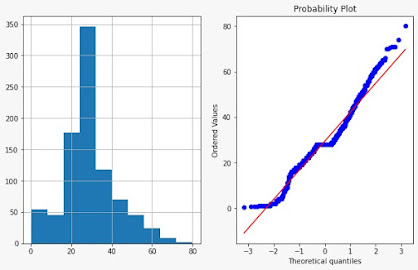Here age is approximately normally distributed, but it's ok we have to study all the transformation techniques and their impact on the feature. so let's move forward.

### Feature Transformation Techniques

There are many techniques for feature transformation. we will study that are most used in almost all problem statements and important from an interview point of view. The techniques that we will study in this article are listed below.

• Logarithmic Transformation
• Square-root Transformation
• Reciprocal Transformation
• Exponential Transformation
• Box-Cos Transformation

let's get started

#### 1) Logarithmic Transformation

This is the simple, most popular, and commonly used transformation type. It has a significant effect on the shape of a variable. The simple formula we have to use is a natural logarithm(log base 10) for each value to make an extremely skewed distribution less skewed.

✅NOTE - The technique can only be applied to strictly positive numbers (Non-zero numbers).

Let's apply this to our selected feature and see the changes in distribution.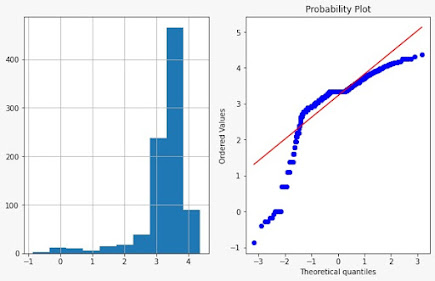Observations

• We can see the significant effect on the shape of a distribution.
• Here, the distribution of shape of slightly disturbed because it was normally distributed only but on other variables, it applies changes significantly.

#### 2) Square-root Transformation

The technique is mostly used and suitable to transform right-skewed distribution. It has an average effect on the shape of the distribution.

NOTE - The technique is only for positive values but the advantage is that we can use it for zero values also.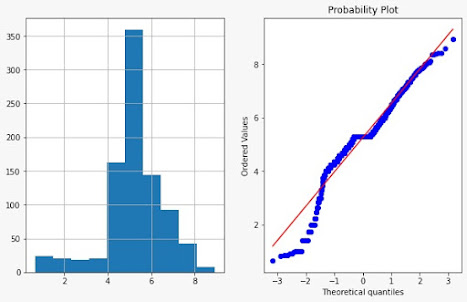we can see how the shape of the distribution is changed.

#### 3) Reciprocal Transformation

It has a radical effect on the shape of distribution which simply means reversing the order among the same sign. It can be used on positive as well on negatively skewed transformation. But cannot be used on zero-valued data.

Formula,   F(X)  =      1
X#### 4) Exponential Transformation

It is also known as square transformation. In this technique, we basically change the data points with their square. The technique is efficient when we have the negative values in our dataset. The advantage of this technique is that we can apply it to all kinds of values. The technique is efficiently useful for the left-skewness.We can see the result of this technique on our variable as well. it is giving a pretty good result.

#### 5) Box-Cox Transformation

Box-Cox transformation is the evolution of the exponential transformation and it is the most successful transformation technique. It basically looks at various exponents instead of trying them manually. By doing so we are searching and evaluating for the best value of Y that most dominates to form normal distribution between -5 to +5.

The Box-Cox transformation is defined as:

T(Y)=(Y exp(λ)−1)/λ

• The y is the response variable and λ is the transformation parameter.
• The exponent here is the term called lambda which varies from -5 to +5, And in the process of searching, it examines each value of lambda and finds the optimal value(which is the best approximation for normal distribution) for our variable.

✅NOTE: It is only applicable for positive numbers only.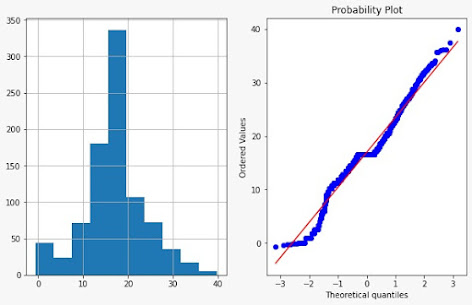we can compare the transformation of Box-Cox and other methods. it is pretty better than every other technique.

👉 You can access the Kaggle Notebook from here and practice all the techniques there if you do not have Jupyter Notebook installed.

## SUMMARY

We got to know the skewness property of the variables in our dataset. we have studied that if the feature is left or right-skewed then how can we transform the feature to a normal distribution using feature transformation techniques. In our upcoming part-4, we will study the feature of various scaling techniques and move forward with each thing required for the feature engineering tasks.

I hope that guys it was easy to take over all the steps and this technique will help you while dealing with any machine learning problem statement.

Thank You! 😊
Keep Learning, Happy Learning